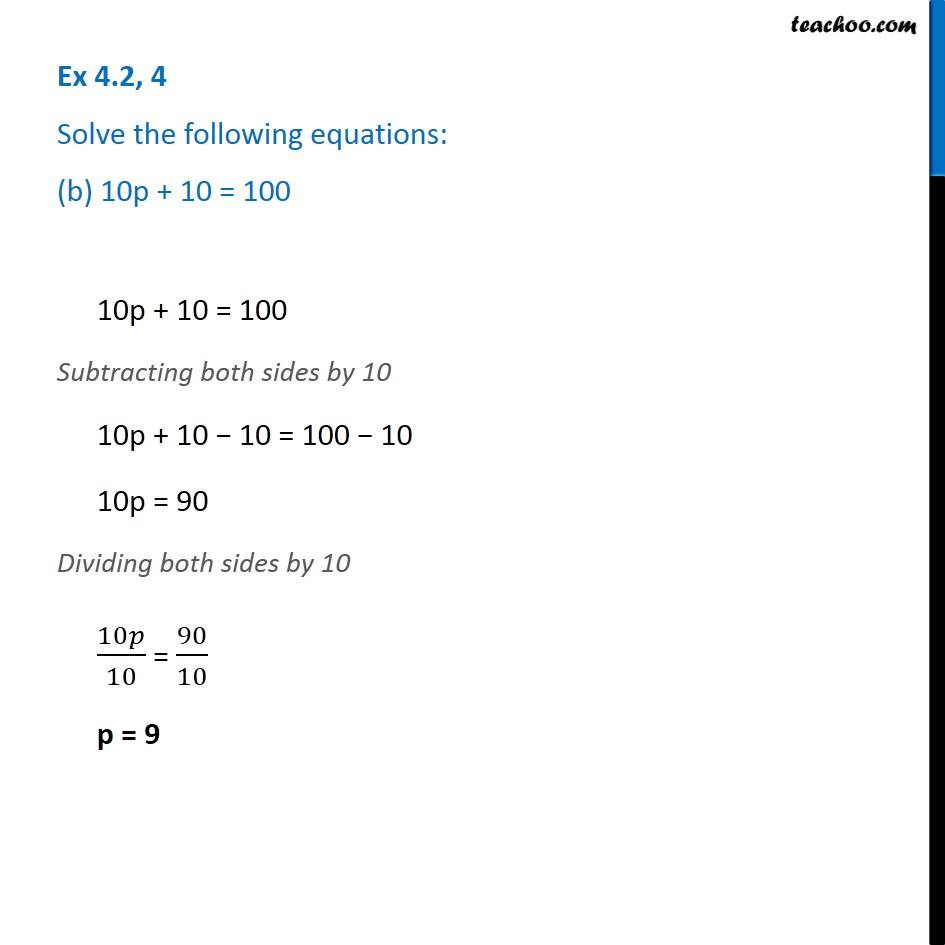1. Chapter 4 Class 7 Simple Equations
2. Serial order wise
3. Ex 4.2

Transcript

Ex 4.2, 4 Solve the following equations: (b) 10p + 10 = 100 10p + 10 = 100 Subtracting both sides by 10 10p + 10 − 10 = 100 − 10 10p = 90 Dividing both sides by 10 10𝑝/10 = 90/10 p = 9